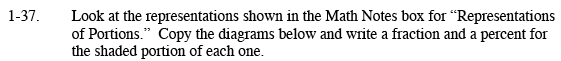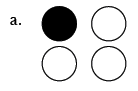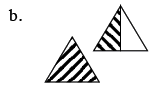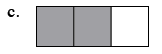### Home > MC2 > Chapter 1 > Lesson 1.1.5 > Problem1-37

1-37.A fraction is a number that represents a part of the whole. How many parts are shaded and how many total parts are there?

The shaded pieces make up the numerator while the total number of pieces makes up the denominator.

To convert from fraction to percent, use standard long division to get the decimal form, and then multiply by 100 or shift the decimal point twice to the right.

For example:

$\frac{3}{20}\rightarrow 0.15\rightarrow 15\%$

$\text{Fraction: }\frac {1}{4}$

Percentage: 25%Think of each triangle as two pieces. Then use the method from part (a).See part (a) and (b).